All the solutions provided in McGraw Hill Math Grade 1 Answer Key PDF Chapter 11 Test as per the latest syllabus guidelines.

Question 1.
Circle the name of the shape.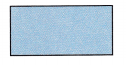circle
rectangle
triangle
Rectangle
Explanation:
A rectangle has 4 sides and 4 corners
Opposite sides are equal.

Question 2.
Circle the name of the solid figure.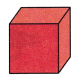cube
cone
cylinder
Cube
Explanation:
A cube has 6 faces and 8 vertices
A faces are in same size.

Question 3.
Color 3 of the 4 equal parts.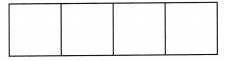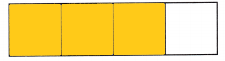Explanation:
The rectangle is divided into 4 equal parts
3 out of 4 is colored.

Question 4.
How many equal parts does the shape have?Is the shape divided into halves or fourths?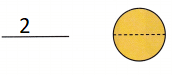Explanation:
The circle is divided in to 2 equal parts.
Line of symmetry a line divides the shapes into 2 equal halves.

Question 5.
Find the shape that matches. Circle it.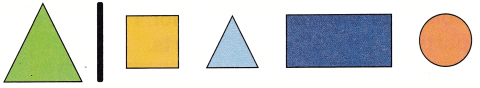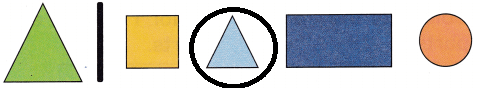Explanation:
The given shape is a triangle so, circled the triangle.

Question 6.
Color the squares.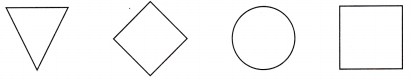Explanation:
In the given shapes there are 2 squares
so, colored the squares.

Question 7.
What shape do you see? Circle the name.rectangle
square
circle
triangle
Square
Explanation:
The face of the cube is a square, a cube has 6 faces.
All the faces are small sizes.

Question 8.
Look at the solid figure. Circle the object that has the same shape.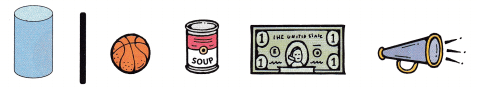Explanation:
The given shape is a cylinder.
so, circled the cylinder shape.

Question 9.
Look at the two red shapes. What new shape can you make? Circle the name of the new shape.square
triangle
cone
circle
Square
Explanation:
The given shapes are rectangles if we join the rectangles it forms a square.

Question 10.
Color 3 of the 4 equal parts.Color 1 of the 2 equal parts.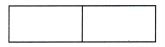Circle the rectangle with the smaller parts. Tell why the parts are smaller.